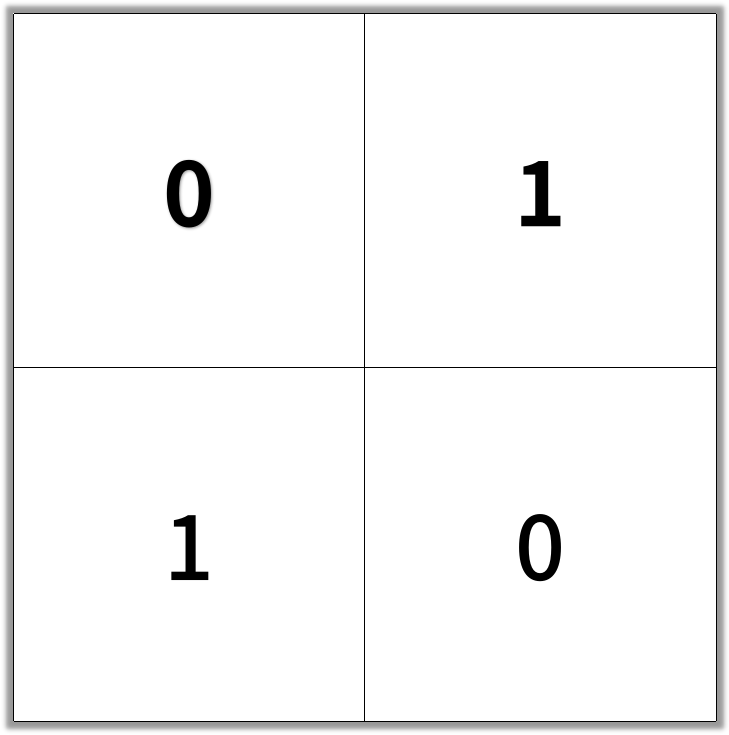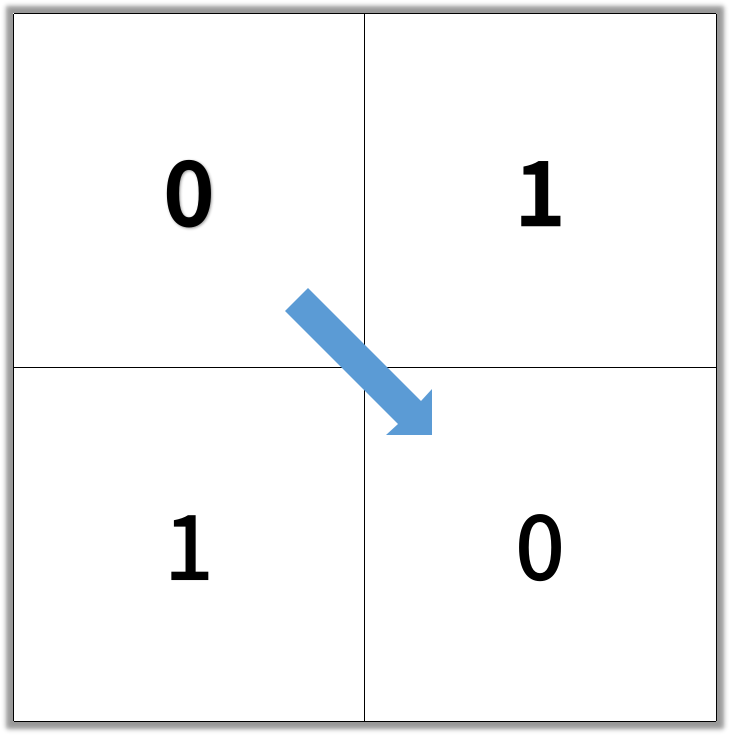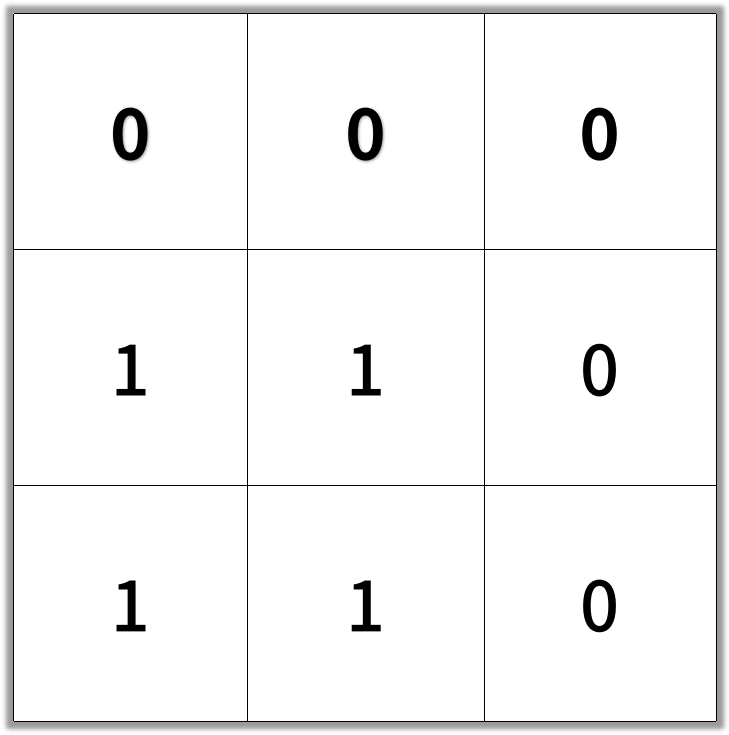## 1091. Shortest Path in Binary Matrix

In an N by N square grid, each cell is either empty (0) or blocked (1).

clear path from top-left to bottom-right has length `k` if and only if it is composed of cells `C_1, C_2, ..., C_k` such that:

• Adjacent cells `C_i` and `C_{i+1}` are connected 8-directionally (ie., they are different and share an edge or corner)
• `C_1` is at location `(0, 0)` (ie. has value `grid`)
• `C_k` is at location `(N-1, N-1)` (ie. has value `grid[N-1][N-1]`)
• If `C_i` is located at `(r, c)`, then `grid[r][c]` is empty (ie. `grid[r][c] == 0`).

Return the length of the shortest such clear path from top-left to bottom-right.  If such a path does not exist, return -1.

Example 1:

```Input: [[0,1],[1,0]]Output: 2```

Example 2:

```Input: [[0,0,0],[1,1,0],[1,1,0]]Output: 4```

Note:

1. `1 <= grid.length == grid.length <= 100`
2. `grid[r][c]` is `0` or `1`

## Rust Solution

``````struct Solution;
use std::collections::VecDeque;

type Point = (usize, usize);

impl Solution {
fn shortest_path_binary_matrix(grid: Vec<Vec<i32>>) -> i32 {
let n = grid.len();
let m = grid.len();
let mut queue: VecDeque<Point> = VecDeque::new();
if grid == 1 || grid[(n - 1) as usize][(m - 1) as usize] == 1 {
return -1;
}
let mut visited: Vec<Vec<bool>> = vec![vec![false; m]; n];
visited = true;
queue.push_back((0, 0));
let mut distance = 0;
let offsets = [
(-1, -1),
(0, -1),
(1, -1),
(-1, 1),
(0, 1),
(1, 1),
(-1, 0),
(1, 0),
];
while !queue.is_empty() {
distance += 1;
let queue_size = queue.len();
for _ in 0..queue_size {
let p = queue.pop_front().unwrap();
if p.0 == n - 1 && p.1 == m - 1 {
return distance;
} else {
for offset in &offsets {
let x = p.0 as i32 + offset.0;
let y = p.1 as i32 + offset.1;
if !(x < 0 || x >= n as i32 || y < 0 || y >= m as i32)
&& grid[x as usize][y as usize] == 0
&& !visited[x as usize][y as usize]
{
visited[x as usize][y as usize] = true;
queue.push_back((x as usize, y as usize));
}
}
}
}
}
-1
}
}

#[test]
fn test() {
let grid = vec_vec_i32![[0, 1], [1, 0]];
let res = 2;
assert_eq!(Solution::shortest_path_binary_matrix(grid), res);
let grid = vec_vec_i32![[0, 0, 0], [1, 1, 0], [1, 1, 0]];
let res = 4;
assert_eq!(Solution::shortest_path_binary_matrix(grid), res);
let grid = vec_vec_i32![[1, 0, 0], [1, 1, 0], [1, 1, 0]];
let res = -1;
assert_eq!(Solution::shortest_path_binary_matrix(grid), res);
let grid = vec_vec_i32![
[0, 1, 0, 0, 0, 0],
[0, 1, 1, 1, 1, 1],
[0, 0, 0, 0, 1, 1],
[0, 1, 0, 0, 0, 1],
[1, 0, 0, 1, 0, 1],
[0, 0, 1, 0, 1, 0]
];
let res = 7;
assert_eq!(Solution::shortest_path_binary_matrix(grid), res);
}
``````

Having problems with this solution? Click here to submit an issue on github.